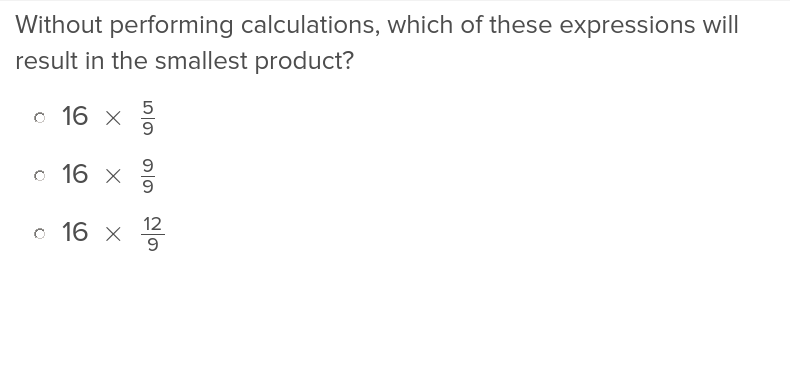Learning Library
Who we are
Guidance

# 5.NF.B.5.A Worksheets, Workbooks, Lesson Plans, and Games

#### CCSS.MATH.CONTENT.5.NF.B.5.A

:
"Comparing the size of a product to the size of one factor on the basis of the size of the other factor, without performing the indicated multiplication."

These exercises can help students practice this Common Core State Standards skill.

## Worksheets

No worksheets found for this common core node.

## Lesson Plans

No plans found for this common core node.

## Workbooks

No workbooks found for this common core node.

## Games

No games found for this common core node.

## ExercisesInterpret Multiplication of Fractions as Scaling
Exercise
Interpret Multiplication of Fractions as Scaling
Introduce your fifth graders to multiplying by fractions with these exercises that help them understand the effect of numerators and denominators of equations.